## Brachistochrone Problem

Find the shape of the Curve down which a bead sliding from rest and Accelerated by gravitywill slip (without friction) from one point to another in the least time. This was one of the earliest problems posed in the Calculus of Variations. The solution, a segment of a Cycloid, was found by Leibniz,L'Hospital,Newton,and the two Bernoullis.

The time to travel from a point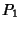to another point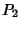is given by the Integral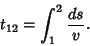(1)

The Velocity at any point is given by a simple application of energy conservationequating kinetic energyto gravitational potential energy,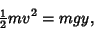(2)

so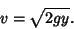(3)

Plugging this into (1) then gives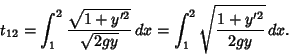(4)

The function to be varied is thus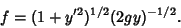(5)

To proceed, one would normally have to apply the full-blown Euler-Lagrange Differential Equation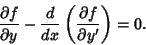(6)

However, the function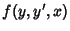is particularly nice sincedoes not appear explicitly. Therefore,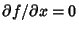, and we can immediately use the Beltrami Identity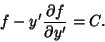(7)

Computing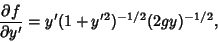(8)

subtracting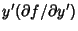from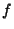, and simplifying then gives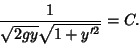(9)

Squaring both sides and rearranging slightly results in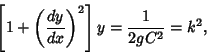(10)

where the square of the old constant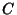has been expressed in terms of a new (Positive) constant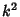. This equation is solved by the parametric equations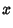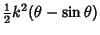(11)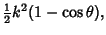(12)

which are--lo and behold--the equations of a Cycloid.

If kinetic frictionis included, the problem can also be solved analytically, although the solution is significantly messier. In that case, terms corresponding to the normal component of weightand the normal component of the Acceleration (present because of path Curvature) must be included. Including both terms requires a constrained variational technique (Ashby et al. 1975), but including the normal component of weight only gives an elementary solution. The Tangent and Normal Vectors are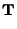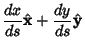(13)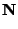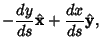(14)

gravityand frictionare then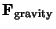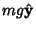(15)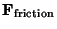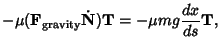(16)

and the components along the curve are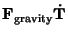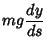(17)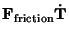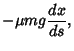(18)

so Newton's Second Lawgives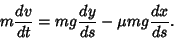(19)

But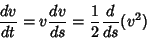(20)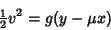(21)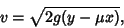(22)

so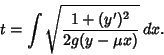(23)

Using the Euler-Lagrange Differential Equation gives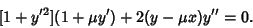(24)

This can be reduced to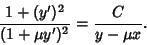(25)

Now letting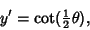(26)

the solution is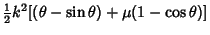(27)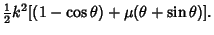(28)

References

Ashby, N.; Brittin, W. E.; Love, W. F.; and Wyss, W. Brachistochrone with Coulomb Friction.'' Amer. J. Phys. 43, 902-905, 1975.

Haws, L. and Kiser, T. Exploring the Brachistochrone Problem.'' Amer. Math. Monthly 102, 328-336, 1995.

Wagon, S. Mathematica in Action. New York: W. H. Freeman, pp. 60-66 and 385-389, 1991.